# Math in Focus Grade 6 Chapter 2 Lesson 2.1 Answer Key Negative Numbers

Go through the Math in Focus Grade 6 Workbook Answer Key Chapter 2 Lesson 2.1 Negative Numbers to finish your assignments.

## Math in Focus Grade 6 Course 1 A Chapter 2 Lesson 2.1 Answer Key Negative Numbers

### Math in Focus Grade 6 Chapter 2 Lesson 2.1 Guided Practice Answer Key

Write a positive or negative number to represent each situation.

Question 1.
36°F below zero
-36°F
Explanation:
The given situation is 36°F below zero. The word ‘below zero’ represents negative sign. The given situation is represented with negative number -36°F.

Question 2.
A debit of $10,540 Answer: -$10,540
Explanation:
The given situation is a debit of $10,540. The word debit is represented with negative sign and credit is represented with positive sign. The given situation is represented with negative number -$10,540.

Question 3.
29,035 feet below sea level
-29,035 feet
Explanation:
The given situation is 29,035 feet below sea level. The word ‘below sea level’ is represented with negative sign. So, the given situation is represented with negative number -29,035 feet.

Question 4.
A gain of 45 yards
45 yards
Explanation:
The given situation is a gain of 45 yards. The word ‘gain’ is represented with positive sign. The word ‘loss’ is represented with negative sign. So, the given situation is represented with positive number 45 yards.

The table shows the elevations of four locations compared to sea level.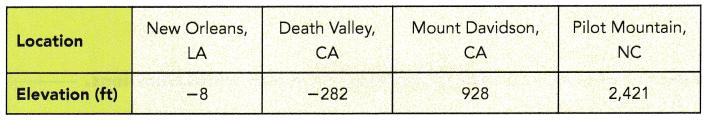Question 5.
New Orleans is 8 feet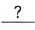sea level.
New Orleans is 8 feet below sea level.

Question 6.
Mount Davidson isfeet above sea level.
Mount Davidson is 928 feet above sea level.

Question 7.
The deepest location among the four locations is.
The deepest location among the four locations is Death Valley.

Question 8.
The highest location among the four locations isThe highest location among the four locations is Pilot Mountain.

Question 9.
The location nearest to sea level is.
The location nearest to sea level is New Orleans.

Copy and complete each inequality using > or <.

Question 10.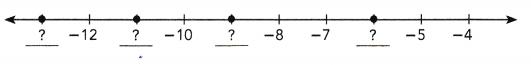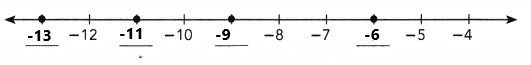-13 < -12 < -11 < -10 < -9 < -8 < -7 < -6 < -5 < -4
Explanation:
The missing numbers on the horizontal number line are -13, -11, -9, -6. On a horizontal number line, the numbers become greater as you move to the right, and less as you move to the left as we can observe in the above image.

Draw a horizontal number line to represent each set of numbers.

Question 11.
-8, -5, -4, -2, 2, 5, 7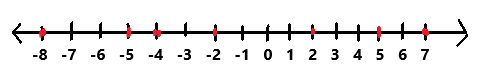The given numbers -8, -5, -4, -2, 2, 5, 7 are represented in the above horizontal number line. On a horizontal number line, the numbers become greater as you move to the right, and less as you move to the left.

Question 12.
-16, -19, -20, -22, -25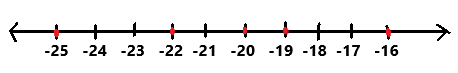The given numbers -16, -19, -20, -22, -25 are represented in the above horizontal number line. On a horizontal number line, the numbers become greater as you move to the right, and less as you move to the left.

Write the opposite of each number.

Question 13.
-14
The opposite number of -14 is 14.

Question 14.
-9
The opposite number of -9 is 9.

Question 15.
-17
The opposite number of -17 is 17.

Question 16.
27
The opposite number of 27 is -27.

Question 17.
-23
The opposite number of -23 is 23.

Question 18.
46
The opposite number of 46 is -46.

Copy and complete each Inequality using > or <.

Question 19.
-15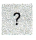-6
-15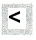-6
Explanation:
The given numbers are -15 and -6. Here we have to compare both the numbers. By comparing the above given numbers the number -15 is less than -6.

Question 20.
-20-23
-20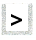-23
The given numbers are -20 and -23. Here we have to compare both the numbers. By comparing the above given numbers the number -20 is greater than -23.

Question 21.
-30-3
-30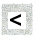-3
The given numbers are -30 and -3. Here we have to compare both the numbers. By comparing the above given numbers the number -30 is less than -3.

Question 22.
-190
-19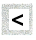0
The given numbers are -19 and 0. Here we have to compare both the numbers. By comparing the above given numbers the number -19 is less than 0.

Question 23.
12-31
12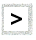-31
The given numbers are 12 and -31. Here we have to compare both the numbers. By comparing the above given numbers the number 12 is greater than -31.

Question 24.
-7546
-75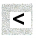46
The given numbers are -75 and 46. Here we have to compare both the numbers. By comparing the above given numbers the number -75 is less than 46.

Hands-On Activity

Materials:

• number cards

Work in pairs. Your teacher will give you and your partner a set of number cards with these numbers on them: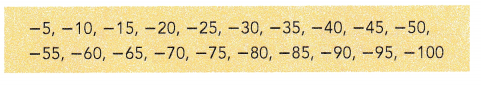Step 1.
Draw a number line and divide it into ten equal intervals. Label the endpoints -100 and 0. Shuffle the cards and place them face down on a flat surface.
Step 2.
Choose one card and turn it over. Mark the number shown on the card on the drawn number line.
Step 3.
Have your partner choose another card and mark the number shown on the number line.
Step 4.
Work together to write a statement of inequality for the two numbers you represented on the number line.
Step 5.
Repeat Step 2 through Step 4 using the other number cards.

Interpret and explain statements of order for positive and negative numbers in real-world situations.

a) The table shows the lowest recorded temperature in Alaska for each month from July through December.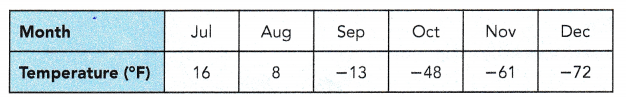September’s lowest recorded temperature of -13°F is lower than August’s lowest recorded temperature of 8°F.
To compare these two temperatures using an inequality, you can write -13°F < 8°F.

b) The table shows the elevations of some natural features.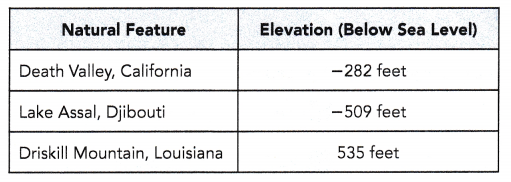The elevation of Death Valley, which is -282 feet, ¡s greater than the elevation of Lake Assai, which is -509 feet.
To compare the two elevations using an inequality, you can write -282 ft > -509 ft.
The elevation of Lake Assai, which is -509 feet, is less than the elevation of Driskill Mountain, which has an elevation of 535 feet.
To compare the two elevations using an inequality, you can write -509 ft < 535 ft.

You can also write the inequalities above as -509ft < -282 ft and 535 ft > -509 ft.Write an inequality for each statement using > or <.

Question 25.
0°C is warmer, than -5°C.
0°C > -5°C
Explanation:
The given statement is 0°C is warmer, than -5°C. Here we have to compare both the temperatures. After comparing the temperatures 0°C is greater than -5°C.

Question 26.
The elevation of the Valdes Peninsula, which is -131 feet, is less than the elevation of the Caspian Sea, which is -92 feet.
-131 feet < -92 feet
Explanation:
The elevation of the Valdes Peninsula is -131 feet.
The elevation of the Caspian Sea is -92 feet.
After comparing both the elevations -131 feet is less than -92 feet.

Write a statement to describe each inequality.

Question 27.
-61 °F < -47°F
The lowest recorded temperature yesterday was -61°F, which is colder than today’s lowest recorded temperature of -47°F.

Question 28. –
520 feet > -893 feet
A dog is standing on a cliff, 520 feet above sea level which is greater than a dolphin which is swimming 893 feet below sea level.

### Math in Focus Course 1A Practice 2.1 Answer Key

Write a positive or negative number to represent each situation.

Question 1.
438°C above zero
438°C
Explanation:
The given situation is 438°C above zero. The word ‘below zero’ represents negative number and ‘above zero’ represents positive number. So the situation is represented with positive number 438°C, which is above zero.

Question 2.
164°F below zero
-164°F
Explanation:
The given situation is 164°F below zero. The word ‘below zero’ represents negative number and ‘above zero’ represents positive number. So the situation is represented with negative number -164°F, which is below zero.

Question 3.
8,327 feet below sea level
-8,327 feet
Explanation:
The given situation is 8,327 feet below sea level. The word ‘below sea level’ represents negative number and ‘above sea level’ represents positive number. So the situation is represented with negative number -8,327 feet, which is below sea level.

Question 4.
12,316 feet above sea level
12,316 feet
Explanation:
The given situation is 12,316 feet above sea level. The word ‘below sea level’ represents negative number and ‘above sea level’ represents positive number. So the situation is represented with positive number 12,316 feet, which is above sea level.

Question 5.
A loss of 20 yards
-20 yards
Explanation:
The given situation is a loss of 20 yards. In general loss is represented with negative number and gain is represented with positive number. So the given situation is represented with negative number -20 yards.

Question 6.
A credit of $3,401 Answer:$3,401
Explanation:
The given situation is a credit of $3,401. In general debit is represented with ‘negative sign’ and credit is represented with ‘positive sign’. So, the given situation is represented with positive number$3,401.

Copy and complete each number line by filling in the missing numbers.

Question 7.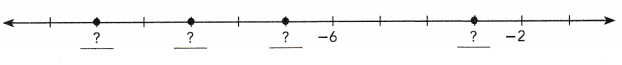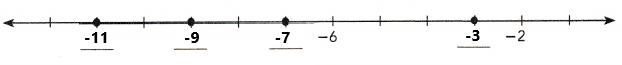Explanation:
The missing numbers are -11, -9, -7, -3. The above number line is filled with the missing numbers as we can observe in the above image.

Question 8.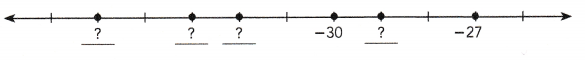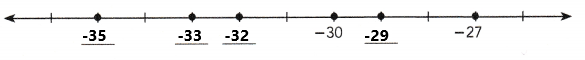Explanation:
The missing numbers are -35, -33, -32, -29. The above number line is filled with the missing numbers as we can observe in the above image.

Write the opposite of each number.

Question 9.
8
The opposite number of 8 is -8.

Question 10.
-5
The opposite number of -5 is 5.

Question 11.
21
The opposite number of 21 is -21.

Question 12.
-29
The opposite number of -29 is 29.

Question 13.
24
The opposite number of 24 is -24.

Question 14.
-106
The opposite number of -106 is 106.

Draw a horizontal number line to represent each set of numbers.

Question 15.
Even negative numbers from -24 to -1o
Even negative numbers from -24 to -1o are -24, -22, -20 ,-18, -16, -14, -12, -10.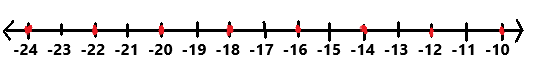Explanation:
The even negative numbers are  -24, -22, -20 ,-18, -16, -14, -12, -10 are represented in the above horizontal number line. On a horizontal number line, the numbers become greater as you move to the right, and less as you move to the left.

Question 16.
The opposites of the whole numbers from 35 to 45
The opposites of the whole numbers from 35 to 45 are -35, -36, -37, -38, -39, -40, -41, -42, -43, -44, -45.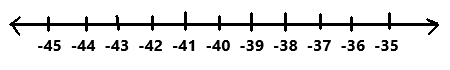Explanation:
The opposites of the whole numbers from 35 to 45 are -35, -36, -37, -38, -39, -40, -41, -42, -43, -44, -45 are represented in the above horizontal number line. On a horizontal number line, the numbers become greater as you move to the right, and less as you move to the left.

Draw a vertical number line to represent each set of numbers.

Question 17.
Odd numbers between -91 and -103
Odd numbers between -91 and -103 are -93, -95, -97, -99, -101.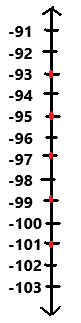Explanation:
The odd numbers between -91 and -103 are -93, -95, -97, -99, -101 are represented in the above vertical number line. On a vertical number line, the numbers become greater as you move up, and lesser as you move down.

Question 18.
Even numbers greater than -6 but less than 12
Even numbers greater than -6 but less than 12 are -4, -2, 0, 2 , 4, 6, 8, 10.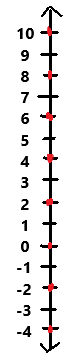Explanation:
Even numbers greater than -6 but less than 12 are -4, -2, 0, 2 , 4, 6, 8, 10 are represented in the above vertical number line. On a vertical number line, the numbers become greater as you move up, and lesser as you move down.

Use the number line to compare each pair of numbers using > or <.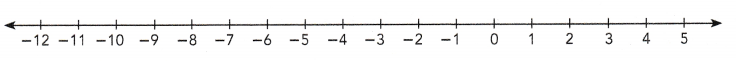Question 19.
-9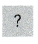-2
-9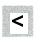-2
Explanation:
In the above horizontal number line, the numbers become greater as you move to the right, and less as you move to the left.
Here -9 is to the left of -2.
So, -9 < -2.

Question 20.
-10-4
-10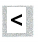-4
Explanation:
In the above horizontal number line, the numbers become greater as you move to the right, and less as you move to the left.
Here -10 is to the left of -4.
So, -10 < -4.

Question 21.
-54
-5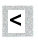4
Explanation:
In the above horizontal number line, the numbers become greater as you move to the right, and less as you move to the left.
Here -5 is to the left of 4.
So, -5 < 4.

Question 22.
2-6
2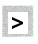-6
Explanation:
In the above horizontal number line, the numbers become greater as you move to the right, and less as you move to the left.
Here 2 is to the right of -6.
So, 2 > -6.

Question 23.
-5-12
-5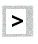-12
Explanation:
In the above horizontal number line, the numbers become greater as you move to the right, and less as you move to the left.
Here -5 is to the right of -12.
So, -5 > -12.

Question 24.
-103
-10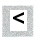3
Explanation:
In the above horizontal number line, the numbers become greater as you move to the right, and less as you move to the left.
Here -10 is to the left of 3.
So, -10 < 3.

Copy and complete each inequality using > or <.

Question 25.
-27-3
-27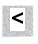-3
Explanation:
The given numbers are -27 and -3. Here we have to compare both the numbers. By comparing the above given numbers the number -27 is less than -3.

Question 26.
-4515
-45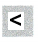15
Explanation:
The given numbers are -45 and 15. Here we have to compare both the numbers. By comparing the above given numbers the number -45 is less than 15.

Question 27.
25-25
25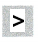-25
Explanation:
The given numbers are 25 and -25. Here we have to compare both the numbers. By comparing the above given numbers the number 25 is greater than -25.

Question 28.
19-15
19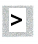-15
Explanation:
The given numbers are 19 and -15. Here we have to compare both the numbers. By comparing the above given numbers the number 19 is greater than -15.

Question 29.
14-16
14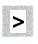-16
Explanation:
The given numbers are 14 and -16. Here we have to compare both the numbers. By comparing the above given numbers the number 14 is greater than -16.

Question 30.
-81-80
-81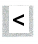-80
Explanation:
The given numbers are -81 and -80. Here we have to compare both the numbers. By comparing the above given numbers the number -81 is less than -80.

Order the numbers in each set from least to greatest.

Question 31.
3, 7, -2, -9, 0, -5
The above given numbers from least to greatest are -9, -5, -2, 0, 3, 7.

Question 32.
-10, 8, 34, -13, 10, -17
The above given numbers from least to greatest are -17, -13, -10, 8, 10, 34.

Order the numbers in each set from greatest to least.

Question 33.
-14, 43, -20, -57, 19, 31
The above given numbers from greatest to least are 43, 31, 19, -14, -20, -57.

Question 34.
98, -101, -76, 125, -92, 113
The above given numbers from greatest to least are 125, 113, 98, -76, -92, -101.

Question 35.
Name two numbers that are each 2 units away from -7. Give the opposites of these two numbers.

Question 36.
Math Journal Is the opposite of a number always negative? Explain
No. The opposite of a negative integer is a positive number. For example, the opposite of -1 is 1.
Explanation:
The opposite of any positive integer is negative. The opposite of any negative integer is positive. Zero is its own opposite.

Question 37.
Write an inequality using > or < for the following statement: -22°C is colder than -4°C.
-22°C < -4°C
Explanation:
The given statement is -22°C is colder than -4°C. Here we have to compare both the temperatures. After comparing the temperatures -22°C is less than -4°C.

Question 38.
Your friend says that the statement 0 < -15 is correct. Explain why the statement is incorrect.
The statement 0 < -15 is incorrect.
-15 is the negative integer.
0 is the positive integer.
Explanation:
Consider a horizontal number line, In that line the numbers become greater as you move to the right, and less as you move to the left.
Here -15 is to the left of 0.
So, -15 < 0.

Question 39.
The elevation of the deepest part of the Pacific Ocean is -36,200 feet. The elevation of the deepest part of the Indian Ocean is -24,442 feet.
Write an inequality to compare the elevations. In which of the two oceans is the deepest part farther from sea level?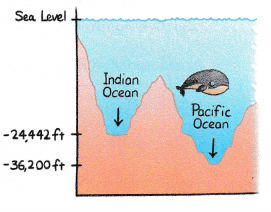The elevation of the deepest part of the Pacific Ocean is -36,200 feet.
The elevation of the deepest part of the Indian Ocean is -24,442 feet.
-36,200 ft < -24,442 ft
Pacific ocean is the deepest part farther from seal level.

Question 40.
The temperature at which a substance boils is called its boiling point. The boiling points of two elements are shown in the table.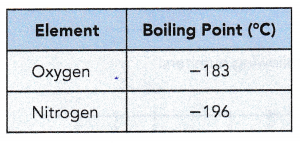Write an inequality to compare the two boiling points. Which element has the greater boiling point?
The boiling point of the oxygen is -183°C.
The boiling point of the Nitrogen is -196 °C.
-183°C > -196 °C
The oxygen has the greatest boiling point with -183°C.

Write a statement to describe each inequality.

Question 41.
-45 feet > -80 feet